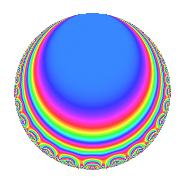# Properties

 Label 4032.2.c.nLevel 4032 Weight 2 Character orbit 4032.c Analytic conductor 32.196 Analytic rank 0 Dimension 4 CM No Inner twists 2

# Related objects

## Newspace parameters

 Level: $$N$$ = $$4032 = 2^{6} \cdot 3^{2} \cdot 7$$ Weight: $$k$$ = $$2$$ Character orbit: $$[\chi]$$ = 4032.c (of order $$2$$ and degree $$1$$)

## Newform invariants

 Self dual: No Analytic conductor: $$32.195682095$$ Analytic rank: $$0$$ Dimension: $$4$$ Coefficient field: $$\Q(\zeta_{12})$$ Coefficient ring: $$\Z[a_1, \ldots, a_{5}]$$ Coefficient ring index: $$2^{5}$$ Sato-Tate group: $\mathrm{SU}(2)[C_{2}]$

## $q$-expansion

Coefficients of the $$q$$-expansion are expressed in terms of a basis $$1,\beta_1,\beta_2,\beta_3$$ for the coefficient ring described below. We also show the integral $$q$$-expansion of the trace form.

 $$f(q)$$ $$=$$ $$q$$ $$-\beta_{1} q^{5}$$ $$+ q^{7}$$ $$+O(q^{10})$$ $$q$$ $$-\beta_{1} q^{5}$$ $$+ q^{7}$$ $$+ 2 \beta_{1} q^{11}$$ $$+ ( -2 \beta_{1} + \beta_{3} ) q^{13}$$ $$-2 q^{17}$$ $$-\beta_{3} q^{19}$$ $$-\beta_{2} q^{23}$$ $$+ ( 1 + \beta_{2} ) q^{25}$$ $$+ ( -\beta_{1} - \beta_{3} ) q^{29}$$ $$+ 4 q^{31}$$ $$-\beta_{1} q^{35}$$ $$+ ( -\beta_{1} - \beta_{3} ) q^{37}$$ $$+ 2 q^{41}$$ $$-2 \beta_{1} q^{43}$$ $$+ ( 4 + 2 \beta_{2} ) q^{47}$$ $$+ q^{49}$$ $$+ ( -3 \beta_{1} + 3 \beta_{3} ) q^{53}$$ $$+ ( 8 - 2 \beta_{2} ) q^{55}$$ $$+ ( -4 \beta_{1} - \beta_{3} ) q^{59}$$ $$+ ( 5 \beta_{1} + 2 \beta_{3} ) q^{61}$$ $$+ ( -8 + 3 \beta_{2} ) q^{65}$$ $$+ ( -2 \beta_{1} + 2 \beta_{3} ) q^{67}$$ $$+ ( 4 + 2 \beta_{2} ) q^{71}$$ $$+ ( 6 - 2 \beta_{2} ) q^{73}$$ $$+ 2 \beta_{1} q^{77}$$ $$+ ( -4 + 2 \beta_{2} ) q^{79}$$ $$+ ( -3 \beta_{1} - 2 \beta_{3} ) q^{83}$$ $$+ 2 \beta_{1} q^{85}$$ $$+ ( 2 + 4 \beta_{2} ) q^{89}$$ $$+ ( -2 \beta_{1} + \beta_{3} ) q^{91}$$ $$-\beta_{2} q^{95}$$ $$+ ( 2 - 2 \beta_{2} ) q^{97}$$ $$+O(q^{100})$$ $$\operatorname{Tr}(f)(q)$$ $$=$$ $$4q$$ $$\mathstrut +\mathstrut 4q^{7}$$ $$\mathstrut +\mathstrut O(q^{10})$$ $$4q$$ $$\mathstrut +\mathstrut 4q^{7}$$ $$\mathstrut -\mathstrut 8q^{17}$$ $$\mathstrut +\mathstrut 4q^{25}$$ $$\mathstrut +\mathstrut 16q^{31}$$ $$\mathstrut +\mathstrut 8q^{41}$$ $$\mathstrut +\mathstrut 16q^{47}$$ $$\mathstrut +\mathstrut 4q^{49}$$ $$\mathstrut +\mathstrut 32q^{55}$$ $$\mathstrut -\mathstrut 32q^{65}$$ $$\mathstrut +\mathstrut 16q^{71}$$ $$\mathstrut +\mathstrut 24q^{73}$$ $$\mathstrut -\mathstrut 16q^{79}$$ $$\mathstrut +\mathstrut 8q^{89}$$ $$\mathstrut +\mathstrut 8q^{97}$$ $$\mathstrut +\mathstrut O(q^{100})$$

Basis of coefficient ring:

 $$\beta_{0}$$ $$=$$ $$1$$ $$\beta_{1}$$ $$=$$ $$-\zeta_{12}^{3} + 2 \zeta_{12}^{2} - 1$$ $$\beta_{2}$$ $$=$$ $$-2 \zeta_{12}^{3} + 4 \zeta_{12}$$ $$\beta_{3}$$ $$=$$ $$3 \zeta_{12}^{3} + 2 \zeta_{12}^{2} - 1$$
 $$1$$ $$=$$ $$\beta_0$$ $$\zeta_{12}$$ $$=$$ $$($$$$\beta_{3}\mathstrut +\mathstrut$$ $$2$$ $$\beta_{2}\mathstrut -\mathstrut$$ $$\beta_{1}$$$$)/8$$ $$\zeta_{12}^{2}$$ $$=$$ $$($$$$\beta_{3}\mathstrut +\mathstrut$$ $$3$$ $$\beta_{1}\mathstrut +\mathstrut$$ $$4$$$$)/8$$ $$\zeta_{12}^{3}$$ $$=$$ $$($$$$\beta_{3}\mathstrut -\mathstrut$$ $$\beta_{1}$$$$)/4$$

## Character Values

We give the values of $$\chi$$ on generators for $$\left(\mathbb{Z}/4032\mathbb{Z}\right)^\times$$.

 $$n$$ $$127$$ $$577$$ $$1793$$ $$3781$$ $$\chi(n)$$ $$1$$ $$1$$ $$1$$ $$-1$$

## Embeddings

For each embedding $$\iota_m$$ of the coefficient field, the values $$\iota_m(a_n)$$ are shown below.

For more information on an embedded modular form you can click on its label.

Label $$\iota_m(\nu)$$ $$a_{2}$$ $$a_{3}$$ $$a_{4}$$ $$a_{5}$$ $$a_{6}$$ $$a_{7}$$ $$a_{8}$$ $$a_{9}$$ $$a_{10}$$
2017.1
 −0.866025 − 0.500000i 0.866025 + 0.500000i 0.866025 − 0.500000i −0.866025 + 0.500000i
0 0 0 2.73205i 0 1.00000 0 0 0
2017.2 0 0 0 0.732051i 0 1.00000 0 0 0
2017.3 0 0 0 0.732051i 0 1.00000 0 0 0
2017.4 0 0 0 2.73205i 0 1.00000 0 0 0
 $$n$$: e.g. 2-40 or 990-1000 Significant digits: Format: Complex embeddings Normalized embeddings Satake parameters Satake angles

## Inner twists

Char. orbit Parity Mult. Self Twist Proved
1.a Even 1 trivial yes
8.b Even 1 yes

## Hecke kernels

This newform can be constructed as the intersection of the kernels of the following linear operators acting on $$S_{2}^{\mathrm{new}}(4032, [\chi])$$:

 $$T_{5}^{4}$$ $$\mathstrut +\mathstrut 8 T_{5}^{2}$$ $$\mathstrut +\mathstrut 4$$ $$T_{11}^{4}$$ $$\mathstrut +\mathstrut 32 T_{11}^{2}$$ $$\mathstrut +\mathstrut 64$$ $$T_{13}^{4}$$ $$\mathstrut +\mathstrut 56 T_{13}^{2}$$ $$\mathstrut +\mathstrut 484$$ $$T_{17}$$ $$\mathstrut +\mathstrut 2$$ $$T_{23}^{2}$$ $$\mathstrut -\mathstrut 12$$ $$T_{31}$$ $$\mathstrut -\mathstrut 4$$